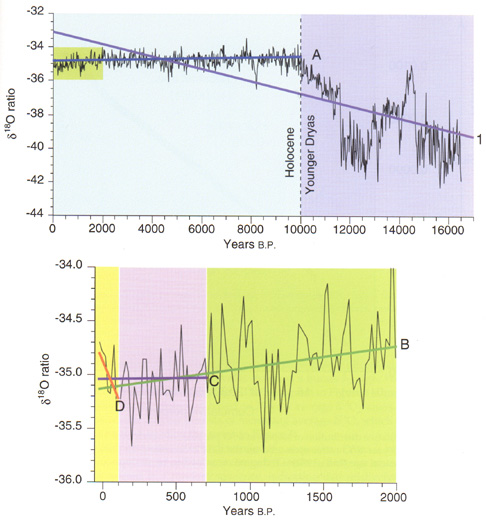Figure 2--Long-term trends in 20-year d18O ratios measured on the GISP2 Greenland ice core. A = Trend since start of Holocene, 10,000 b.p. Slope = -0.000024**. B = Trend since start of Christian era, 2000 b.p. Slope = -0.000196**. C = Trend since start of Little Ice Age, 700 b.p. Slope = -0.000067 NS. D = Trend since start of industrial revolution, 100 b.p. Slope = +0.003518 NS. Positive slope indicates rising temperatures. ** = highly significant (p=0.01), NS = not significant.